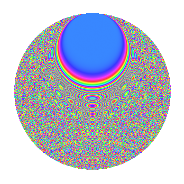# Properties

 Label 1512.2.egLevel 1512 Weight 2 Character orbit eg Rep. character $$\chi_{1512}(155,\cdot)$$ Character field $$\Q(\zeta_{18})$$ Dimension 1296 Sturm bound 576

# Related objects

## Defining parameters

 Level: $$N$$ = $$1512 = 2^{3} \cdot 3^{3} \cdot 7$$ Weight: $$k$$ = $$2$$ Character orbit: $$[\chi]$$ = 1512.eg (of order $$18$$ and degree $$6$$) Character conductor: $$\operatorname{cond}(\chi)$$ = $$216$$ Character field: $$\Q(\zeta_{18})$$ Sturm bound: $$576$$

## Dimensions

The following table gives the dimensions of various subspaces of $$M_{2}(1512, [\chi])$$.

Total New Old
Modular forms 1752 1296 456
Cusp forms 1704 1296 408
Eisenstein series 48 0 48

## Trace form

 $$1296q + O(q^{10})$$ $$1296q + 18q^{12} - 42q^{18} + 42q^{20} + 48q^{30} + 30q^{32} + 24q^{33} - 24q^{41} + 30q^{42} + 144q^{44} + 18q^{48} - 156q^{50} - 54q^{52} + 54q^{58} + 72q^{59} + 126q^{60} - 186q^{66} - 78q^{68} - 42q^{72} - 84q^{74} + 168q^{75} - 156q^{78} - 30q^{86} - 198q^{90} + 114q^{92} + 54q^{94} - 156q^{96} + O(q^{100})$$

## Decomposition of $$S_{2}^{\mathrm{new}}(1512, [\chi])$$ into newform subspaces

The newforms in this space have not yet been added to the LMFDB.

## Decomposition of $$S_{2}^{\mathrm{old}}(1512, [\chi])$$ into lower level spaces

$$S_{2}^{\mathrm{old}}(1512, [\chi]) \cong$$ $$S_{2}^{\mathrm{new}}(216, [\chi])$$$$^{\oplus 2}$$

## Hecke Characteristic Polynomials

There are no characteristic polynomials of Hecke operators in the database